Open in App
Not now

# Class 10 RD Sharma Solutions – Chapter 14 Coordinate Geometry – Exercise 14.3 | Set 3

• Last Updated : 02 Nov, 2022

### Question 41. Three consecutive vertices of a parallelogram are (-2, -1), (1, 0) and (4, 3). Find the fourth vertex.

Solution:

Let the coordinates of three vertices are A (-2, -1), B (1, 0), and C (4, 3)

And let the diagonals AC and BD bisect each other at O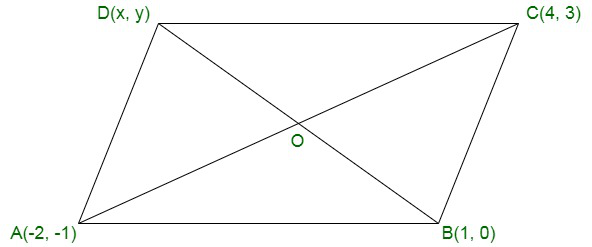As O is the mid-point of AC

Therefore,

Vertices of O will beAssume coordinates of the forth vertex D be (x, y)

As O is the mid-point of BD

Thus, coordinates of O will beTherefore(1 + x)/2 = 1

1 + x = 2

x = 1

and

y/2 = 1

y = 2

Hence, the co-ordinates of D will be (1, 2).

### Question 42. The points (3, -4) and (-6, 2) are the extremities of a diagonal of a parallelogram. If the third vertex is (-1, -3). Find the co-ordinates of the fourth vertex.

Solution:

Assume the edges of a diagonal AC of a parallelogram ABCD are A (3, -4) and C (-6, 2)

Consider AC and BD bisect each other at O.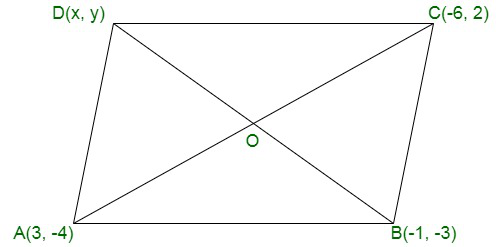Therefore,

Mid-point of AC will beAssume the fourth vertex of the parallelogram be (x, y)

Therefore,

Mid-point of BD will be-1 + x = -3

x = -2

and

(-3 + y)/2 = -1

-3 + y = -2

y = -2 + 3 = 1

Hence, the coordinates of D are (-2, 1).

### Question 43. If the co-ordinates of the mid-points of the sides of a triangle are (1, 1), (2, -3), and (3, 4), find the vertices of the triangle.

Solution:

Assume A (x1, y1), B (x2, y2) and C (x3, y3) be the vertices of the ∆ABC

D, E and F are the mid-points of BC, CA and AB respectively such that their co-ordinates are D (1, 1), E (2, -3) and F (3, 4)

D is mid-point of BC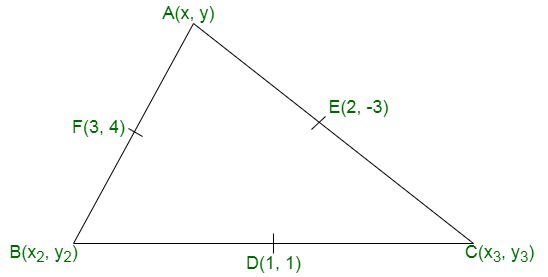Therefore,x2 + x3 = 2

andy2 + y3 = 2

Similarly, E is the mid-point of AC

Therefore,x3 + x1 = 4

andy3 + y1 = -6

And

F is the mid-point of AB

Therefore,x2 + x1 = 6

andy2 + y1 = 8

Now,

x1 + x2 = 6  …………..(i)

x2 + x3 = 2  ……………(ii)

x3 + x1 = 4  …………….(iii)

2(x1 + x2 + x3) = 12

x1 + x2 + x3 = 6  …………(iv)

On subtracting (ii), (iii) and (i) from (iv), we get

x1 = 4, x2 = 2, x3 = 0

Similarly

y1 + y2 = 8  ……….(v)

y2 + y3 = 2  ……….(vi)

y3 + y1 = -6  ………(vii)

2(y1 + y2 + y3) = 4

y1 + y2 + y3 = 2  ………(viii)

On subtracting (vi), (vii) and (v) from (viii), we get

y1 = 0

y2 = 8

y3 = -6

Hence, the vertices of ∆ABC are A (4, 0), B(2, 8), C(0, -6)

### Question 44. Determine the ratio in which the straight line x – y – 2 = 0 divides the line segment joining (3, -1) and (8, 9).

Solution:

Assume the straight line x – y – 2 = 0 divides the line segment joining the points (3, -1), (8, 9) in the ratio m : n

Co-ordinates of the point will beandThis point (x, y) lies on the line on the line x – y – 2 = 0

⇒ (8m + 3n) – (9m – n) – 2(m + n) = 0

⇒ 8m + 3n – 9m + n – 2m – 2n = 0

⇒ -3m + 2n = 0

⇒ 2n = 3mHence, the ratio = 2 : 3 internally

### Question 45. Three vertices of a parallelogram are (a + b, a – b), (2 a + b, 2a – b), (a – b, a + b). Find the fourth vertex.

Solution:

In parallelogram ABCD co-ordinates are of A (a + b, a – b), B (2a + b, 2a – b), C (a – b, a + b)

Assume coordinates of D be (x, y)

Join diagonal AC and BD

Which bisect each other at O

O is the mid-point of AC as well as BD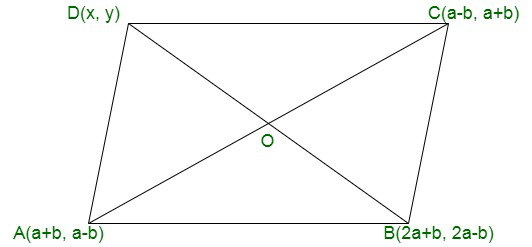If O is the mid-point of AC, Then its coordinates will beand

If O is mid-point of BD, then coordinates will bex + 2a + b = 2a

x = 2a – 2a – b = -b

andy + 2a – b = 2a

y = 2a – 2a + b = b

Hence, the coordinates of D will be (-b, b).

### Question 46. If two vertices of a parallelogram are (3, 2), (-1, 0) and the diagonals cut at (2, -5), find the other vertices of the parallelogram.

Solution:

Two vertices of a parallelogram ABCD are A (3,2), and B (-1, 0) and its diagonals bisect each other at O (2, -5)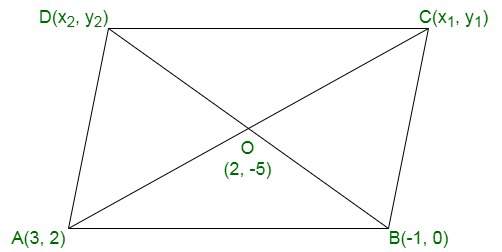Assume the coordinates of C be (x1, y1) and of D be (x2, y2)

If O is the mid-point of AC, then3 + x1 = 4

x1 = 4 – 3 = 1

andand2 + y1 = -10

y1 = -10 – 2 = -12

Therefore, the coordinates of C will be (1, -12)

Again if O is mid-point of BD then-1 + x2 = 4

x2 = 4 + 1 = 5

andy2 = -10

Hence, the coordinates of D will be (5, -10).

### Question 47. If the coordinates of the mid-points of the sides of a triangle ar6 (3, 4), (4, 6), and (5, 7), find its vertices.

Solution:

The coordinates of the mid-points of the sides BC, CA and AB are D (3, 4), E (4, 6) and F (5, 7) of the ∆ABC.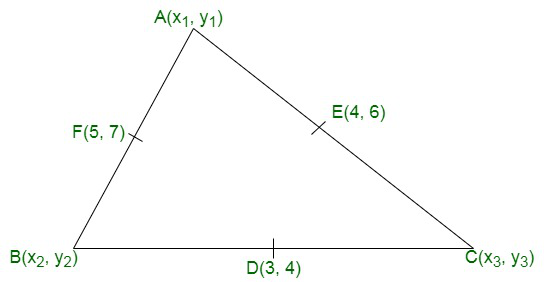Assume the coordinates of the vertices of the triangle be A (x,1 y1), B (x2, y2), and C (x3, y3).

Now the coordinates of D will bex2 + x3 = 6

and

y2 + y3 = 4

y2 + y3 = 8

Similarly, the coordinates of E will bex3 + x1 = 8

andandy1 + y2 = 14

Now,

x2 + x3 = 6  …….(i)

x3 + x1 = 8  ……..(ii)

x1 + x2 = 10  ……..(iii)

2(x1 + x2 + x3) = 24

⇒ x1 + x2 + x3 = 24/2 = 12  ………..(iv)

On subtracting each from (iv),

We will get

x1 = 6, x2 = 4 and x3 = 2

Similarly,

y2 + y3 = 8   ……..(v)

y3 + y1 = 12  ………(vi)

y1 + y2 = 14   ……..(vii)

2(y1 + y2 + y3) = 34

⇒ y1 + y2 + y3 = 34/2  = 17  ……..(viii)

On subtracting each from (viii),

We will get

y1 = 9

y2 = 5

y3 = 3

Hence, the coordinates will be of A (6, 9), B (4, 5) and C (2, 3)

### Question 48. The line segment joining the points P (3, 3) and Q (6, -6) is trisected at the points A and B such that A is nearer to P. If A also lies on the line given by 2x+ y + k =0, find the value of k.

Solution:

Two points A and B trisect the line segment joining the points P (3, 3) and Q (6, -6) and A is nearer to P and A lies also on the line 2x + y + k = 0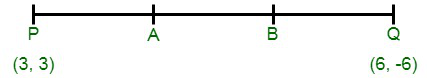Now,

A divides the line segment PQ in the ratio of 1 : 2

i.e.,

PA = AQ = 1 : 2

Assume coordinates of A be (x, y), thenandTherefore,

Coordinates of A are (4, 0)

As A lies on the line 2x + y + k = 0

Hence,

It will satisfy it

2 × 4 + 0 + k = 0

8 + k = 0

k = -8

### Question 49. If three consecutive vertices of a parallelogram are (1, -2), (3, 6), and (5, 10), find its fourth vertex.

Solution:

A (1, -2), B (3, 6) and C (5, 10) are the three consecutive vertices of the parallelogram ABCD

Assume (x, y) be its fourth vertex

AC and BD are its diagonals which bisect each other at O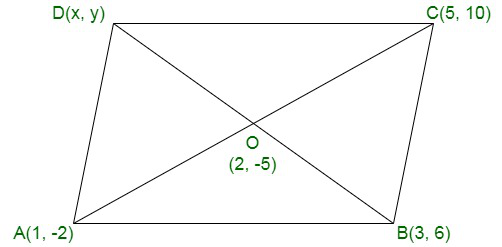As O is the mid-point of AC

Therefore,

Coordinates of O will beOn comparing,

3 + x = 3

3 + x = 6

x = 3

and

(6 + y)/2 = 4

6 + y = 8

y = 2

Hence, the coordinates of fourth vertex D are (3, 2).

### Question 50. If the points A (a, -11), B (5, b), C (2, 15), and D (1, 1) are the vertices of a parallelogram ABCD, find the values of a and b.

Solution:

A (a, -11), B (5, b), C (2, 15) and D (1, 1) are the vertices of a parallelogram ABCD

Diagonals AC and BD bisect each other at O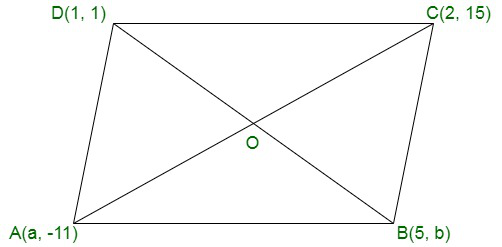As O is the mid-point of AC

Therefore,

Coordinates of O will beSimilarly, O is the mid-point of BD also

Therefore,

Coordinates of O will be

(2 + a)/2 = 3

b + 1 = 4

b = 3

Hence,

a = 4

b = 3

### Question 51. If the co-ordinates of the mid-points of the sides of a triangle be (3, -2), (-3, 1), and (4, -3), then find the coordinates of its vertices.

Solution:

In a ∆ABC,

D, E and F are the mid-points of the sides BC, CA and AB respectively and co-ordinates of D, E and F are (3, -2), (-3, 1) and (4, -3) respectively.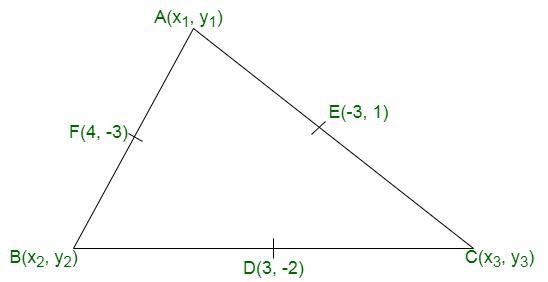Assume the coordinates of A are (x1, y1), of B are (x2, y2) and of C are (x3, y3)

Now,

As D is the mid-point BC

Therefore,andAs E is the mid-point of CA

Therefore,andand F is the mid-point of AB

Therefore,andNow,

x2 + x3 = 6  ……..(i)

x3 + x1 = -6  ……..(ii)

x1 + x2 = 8   ………(iii)

2(x1 + x2 + x3) = 8

⇒ x1 + x2 + x3 = 4   …….(iv)

On subtracting from (iv) we get

x1 = -2,

x2 = 10,

x3 = -4

and

y2 + y3 = -4   …….(v)

y3 + y1 = 2   …….(vi)

y1 + y2 = -6  ………(vii)

2(y1 + y2 + y3) = -8

⇒ y1 + y2 + y3 = -4   ……..(viii)

On subtracting from (viii) we get

y1 = 0,

y2 = -6,

y3 = 2

Hence, the coordinates of A are (-2, 0) of B are (10, -6) and of C (-4, 2).

### Question 52. The line segment joining the points (3, -4) and (1, 2) is trisected at the points P and Q. If the co-ordinates of P and Q are (p, -2) and (53 , q) respectively, find the values of p and q.

Solution:

Assume line AB whose ends points are A (3, -4) and B (1, 2)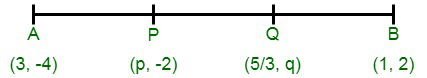Coordinates of P and Q which trisect AB are P (p, -2) and QNow,

As P divides AB in the ratio 1 : 2

Therefore,

Coordinates of P will beSimilarly, Q divides AB in the ratio 2 : 1Hence,

p = 7/3, q = 0

### Question 53. The line joining the points(2, 1), (5, -8) is trisected at the points P and Q. If point P lies on the line 2x – y + k = 0, find the value of k.

Solution:

Points A (2, 1), and B (5, -8) are the ends points of the line segment AB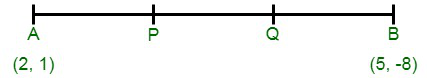Points P and Q trisect it and P lies on the line 2x – y + k = 0

As P divides AB in the ratio of 1 : 2

Therefore,

Coordinates of P will beTherefore,

Coordinates of P are (3, -2)

As it lies on 2x – y + k = 0

Therefore,

It will satisfy it

2 × 3 – (-2) + k = 0

⇒ 6 + 2 + k = 0

⇒ 8 + k = 0

⇒ k = -8

### Question 54. A (4, 2), B (6, 5), and C (1, 4) are the vertices of ∆ABC,

(i) The median from A meets BC in D. Find the coordinates of the point D.

(ii) Find the coordinates of point P on AD such that AP : PD = 2 : 1.

(iii) Find the coordinates of the points Q and R on medians BE and CF respectively such that BQ : QE = 2 : 1 and CR : RF = 2 : 1.

(iv) What do you observe?

Solution:

In ∆ABC, co-ordinates of A (4, 2) of (6, 5) and of (1, 4) and AD is BE and CF are the medians

such that D, E and F are the mid-points of the sides BC, CA and AB respectively

P is a point on AD such that AP : PD = 2 : 1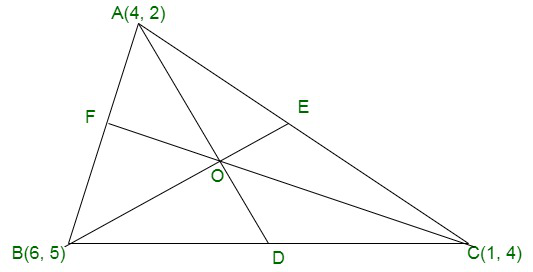(i) Now coordinates of D will be(ii) As P divides AD in the ratio of 2 : 3

Therefore,

Coordinates of P will beThus,

Coordinates of P are(iii) As E and F are the mid-point if CA and AB respectively

Coordinates of E will beand of F will beAs Q and R divides BE and CF in such a way that BQ : QE = 2 : 1 and CR : RF = 2 : 1

Therefore,

Coordinates of Q will beandi.e., Q1 is (11/3, 11/3)

and similarly the coordinates of R will beandR is (11/3, 11/3)

(iv) We can see that coordinates of P, Q and R are same

i.e., P, Q and R coincides each other.

Medians of the sides of a triangle pass through the same point which is called the centroid of the triangle.

### Question 55. If the points A (6, 1), B (8, 2), C (9, 4), and D (k, p) are the vertices of a parallelogram taken in order, then find the values of k and p.

Solution:

The diagonals of a parallelogram bisect each other

O is the mid-point of AC and also of BD

O is the mid-point of AC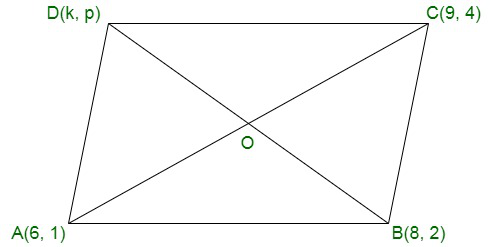Therefore,

Coordinates of O will beAs O is also the mid-point of BD

Therefore,and⇒ 8 + k = 15

⇒ k = 15 – 8 = 7

and

⇒ 2 + p = 5

⇒ p = 5 – 2 = 3

Hence,

k = 7, p = 3

### Question 56. A point P divides the line segment joining the points A (3, -5) and B (-4, 8) such that. If P lies on the line x + y = 0, then find the value of k.

Solution:

Point P divides the line segment by joining the points A (3, -5) and B (-4, 8)

Such that⇒ AP : PB = k : 1

Assume coordinates of P be (x, y), thenandAs x + y = 0

Therefore,4k – 2 = 0

4k = 2

k = 2/4

k = 1/2

### Question 57. The mid-point P of the line segment joining the points A (-10, 4) and B (-2, 0) lies on the line segment joining the points C (-9, -4) and D (-4, y). Find the ratio in which P divides CD. Also, find the value of y.

Solution:

P is the mid-point of line segment joining the points A (-10, 4) and B (-2, 0)

Coordinates of P will beP lies on CD also,

Let P divides C (9, 4) and D (-4, y) in the ratio m1 : m2

Therefore,⇒ -6m1 – 6m2 = -4m1 – 9m2

⇒ -6m1 + 4m1 = -9m2 + 6m2

⇒ -2m1 = -3m2

⇒Therefore,

m1 : m2 = 3 : 2

and⇒ 10 = 3y – 8x

⇒ 3y = 10 + 8 = 18

⇒ y = 18/3 = 6

⇒ y = 6

### Question 58. If the point C (-1, 2) divides internally the line segment joining the points A (2, 5) and B (x, y) in the ratio 3 : 4, find the value of x2 + y2.

Solution:

As we know that if a point (x, y) divides the line segment joining

the points (x1, y1) and (x,2 y2) in the ration m : n, thenandNow here, C (-1, 2) divides the line segment joining A (2, 5) and B (x, y) in the ratio of 3 : 4

Now,and⇒ 3x + 8 = -7

and

⇒ 20 – 3y = 14

⇒ x = -5

and

⇒ y = 2

Now,

x2 + y2 = (-5)2 + (2)2

x2 + y2 = 25 + 4 = 29

### Question 59. ABCD is a parallelogram with vertices A (x1, y1), B (x2, y2), and C (x3, y3). Find the coordinates of the fourth vertex D in terms of x1, x2, x3, y1, y2, and y3

Solution:

Assume the coordinates of D be (x, y). We know that diagonals of a parallelogram bisect each other.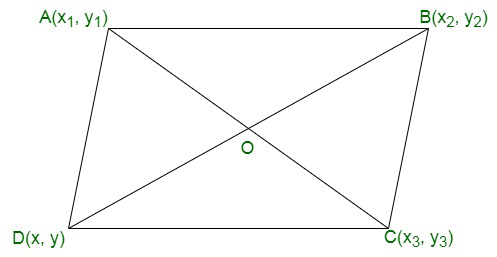Hence,

Mid-point of AC = Mid-point of BDi.e., x1 + x3 = x2 + x and y1 + y3 = y2 + y

i.e, x1 + x3 – x2 = x and y1 + y3 + y2 = y

Hence, the coordinates of D are (x1 + x3 – x2, y1 + y3 + y2)

### Question 60. The points A (x1, y1), B (x2, y2), and C (x3, y3) are the vertices of ∆ABC.

(i) The median from A meets BC at D. Find the coordinates of the point D.

(ii) Find the coordinates of the point P on AD such that AP : PD = 2 : 1.

(iii) Find the points of coordinates Q and R on medians BE and CF respectively such that BQ : QE = 2 : 1 and CR : RF = 2 : 1.

(iv) What are the coordinates of the centroid of the triangle ABC?

Solution:

Given: The points A (x1, y1), B (x2, y2) and C (x3, y3) are the vertices of ∆ABC.

(i) We know that, the median bisect the line segment into two equal parts i.e., here D is the mid-point of BC.

Therefore,

Coordinates of mid-point of BC =D =(ii) Assume the coordinates of a point P be (x, y)

Given that, the point P (x, y), divide the line joining A (x1, y1) andin the ration of 2 : 1,

Then the coordinates of P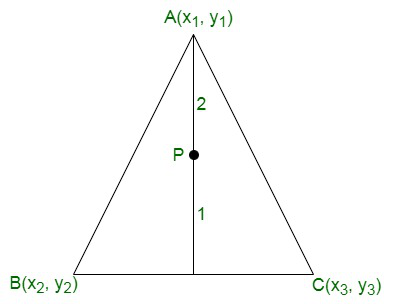Using internal section formula, we getTherefore,

Required coordinates of points P =(iii) Assume the coordinates of a point Q be (p, q)

Given: The point Q (p, q) divide the line joining B(x2, y2) and EIn the ratio of 2 : 1

Then the coordinates of Q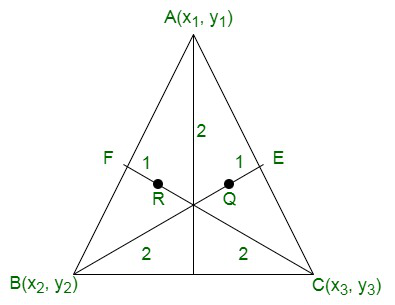[Since, BE is the median of side CA. So, BE divides AC into two equal parts]

Therefore,

Mid-point of AC = Coordinates of E

⇒ E =So, the required coordinate of point Q =Now, let us considered that the coordinates of a point E be (α, β).

It is given that, the point R (α, β), divide the line joining C (x3, y3) andin the ration 2 : 1,

So, the coordinates of R beHere, CF is the median of side AB, hence CF divides AB into two equal parts

Hence,

Mid-point of AB = Coordinates of CF

⇒ FSo, required coordinate of point R(iv) Coordinate of the centroid of the ∆ABC = (sum of abscissa of all vertices/3, sum of all vertices/2)My Personal Notes arrow_drop_up